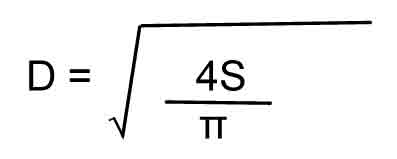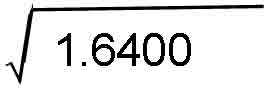# Calculate the Diameter of a Cable

The following formulae can be used to calculate the approximate diameter of a multi-strand cable where both the diameter of an individual strand and the strand-count are known:

s =  (πd2) / 4 (to get the area of a single strand)

S   = n.s (to get the approximate area of a defined number of strands)(to get the approximate diameter of a defined number of strands)

 where s = area of a single strand d = diameter of a single strand D = diameter of a defined number of strands π = 3.14 S = area of defined number of strands n = number of strands

As an example, let's assume we want to know the approximate diameter for a multi-strand cable having 41 strands of 0.20mm wire.

First we need to calculate the area of a single strand:

0.20 x 0.20 = 0.04

0.04 × 3.14 = 0.1256

0.1256 4 = 0.0314

The approximate area for 41 strands is then found:

41 × 0.0314 = 1.2874

Now knowing the approximate area, we can get the approximate diameter by using the formula:(4 × 1.2874)3.14 = 1.6400= 1.2806   (1.3mm Diameter)